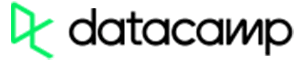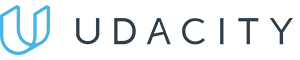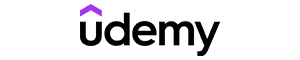Code has been added to clipboard!

# Discover JavaScript Number: JavaScript Numbers Explained

Published Aug 8, 2017
Updated Oct 1, 2019

This tutorial explains what is a number in JavaScript. As you go through it, you'll understand how to write numbers, including JavaScript integers and decimals. You will learn about binary, octal, and hexadecimal numbers, JavaScript infinity and NaN.

The tutorial will cover what JavaScript number precision is as well as number properties. Make sure you fully understand them before learning about number methods.

## JavaScript Number: Main Tips

• There is only one type of number in JavaScript.
• Numbers in JavaScript may be written with or without decimals.
• JavaScript number can hold up to 17 digits after the decimal point until it loses preciseness.
• In JavaScript, methods and properties are accessible to numbers, since they are treated as objects when executing.

## Number Format

All numbers in JavaScript are floating point numbers, unlike in other programming languages, which feature multiple types of numbers. Following the international IEEE 754 standard, all Javascript numbers are double precision floating point numbers.

In this format, numbers are stored in 64 bits, and the fraction number is stored in bits 0 to 51. The exponent is stored in bits 52 to 62, and lastly the sign in the bit 63.

Value (aka Fraction/Mantissa) Exponent Sign
52 bits (0-51) 11 bits (52-62) 1 bit (63)

Extremely large or small numbers may be written using scientific (exponential) notation. It means that some numbers can be transformed into letters for a shorter and neater looking code.

Example
``````var a = 124e5;    // 12400000
var b = 124e-6;   // 0.000124``````

## Precision

Numbers in JavaScript can be written with or without decimals. It is up to you to decide:

Example
``````var a = 21.00;    // number with decimals
var b = 12;       // number without decimals``````

Although the maximum number of digits held after the decimal point is seventeen, keep in mind that at that point JavaScript numbers are not very precise. They are only accurate up to fifteen digits after the decimal point:

Example
``````var a = 999999999999999;   // a will be 999999999999999
var b = 9999999999999999;  // b will be 10000000000000000``````

Look at the example below to see how floating point arithmetics can not always be 100% accurate when the number of digits after the decimal point exceeds fifteen:

Example
``var a = 0.2 + 0.1; // a will return 0.30000000000000004``

To solve the problem in the editor example above, most developers use multiplying and dividing for precise and neat calculations with JavaScript numbers.

Example
``var a = (0.2 * 10 + 0.1 * 10) / 10; // a will return 0.3``

Numeric constants in JavaScript are interpreted as hexadecimals if they are preceded by 0x.

• Numbers (0, 1, 2, 3, 4, 5, 6, 7, 8, 9) are extended to 16 digits.
• Number 9 is preceded by A, B, C, D, E, F.
• A = 10
• B = 11
• C = 12
• D = 13
• E = 14
• F = 15
• Numbers (and letters) are multiplied and the result appears.

This is how it looks in real life:

Example
``var a = 0xFF;``

JavaScript will display numbers as base 10 decimals by default (meaning - 0, 1, 2, 3, 5, 6, 7, 8, 9). However, it is possible to use the `toString()` method for outputting JavaScript numbers as base 2 (binary), base 8 (octal), or base 16 (hex).

Example
``````var a = 321;
a.toString(16);
a.toString(8);
a.toString(2);``````

Note: never write JavaScript numbers with a leading zero, since certain JavaScript versions may interpret them as octal (base 8)!Pros
• Easy to use with a learn-by-doing approach
• Offers quality content
• Gamified in-browser coding experience
• The price matches the quality
• Suitable for learners ranging from beginner to advanced
Main Features
• Free certificates of completion
• Focused on data science skills
• Flexible learning timetablePros
• Simplistic design (no unnecessary information)
• High-quality courses (even the free ones)
• Variety of features
Main Features
• Nanodegree programs
• Suitable for enterprises
• Paid Certificates of completionPros
• Easy to navigate
• No technical issues
• Seems to care about its users
Main Features
• Huge variety of courses
• 30-day refund policy
• Free certificates of completion

## Infinity

JavaScript `Infinity` or `-Infinity` (positive and negative infinity) is the value that is returned by the program when you calculate past the largest possible precise number while using JavaScript.

The largest precise number JavaScript can return is ± 9007199254740991.

Example
``````var a = 4;
while (a != Infinity) {     // executes until infinity (highest possible number is reached
a = a * a;
}``````

However, not only maximum numbers will generate JavaScript `Infinity`. Keep in mind that dividing by 0 (zero) will also generate `Infinity`:

Example
``````var a =  4 / 0;
var b = -4 / 0;``````

`typeOf Infinity` returns a JavaScript number definition:

Example
``typeof Infinity;``

## NaN - Not a Number

If you try to perform arithmetic tasks with non-numeric strings, it will result in `NaN` (Not a Number), indicating that the selected value is not a number, but rather something else.

Example
``var a = 2 / "Car";``

However, in case the string does contain a numeric value, the result is still going to be a number.

Example
``var a = 2 / "2";``

The global JavaScript `isNaN()` function can be used to find out whether a value is a number JavaScript can display, or not:

Example
``````var a = 2 / "Car";
isNaN(a);``````

Note: you should look out for NaN JavaScript. Using NaN in a mathematical operation results in another NaN.

Check out an example of using NaN JavaScript in mathematical operations:

Example
``````var a = NaN;
var b = 4;
var c = a + b;``````

The result may also be concatenation (strings being joined end-to-end):

Example
``````var a = NaN;
var b = "4";
var c = a + b;``````

`typeOf(NaN)` returns a JavaScript number definition:

Example
``typeof NaN;``

Note: Keep in mind that string + string = concatenation, while number + number = addition.

## Numbers As Objects

By default JavaScript numbers are all primitive values that are created from literals: `var x = 321`. However, numbers may also be defined as objects using the keyword `new`. It looks like this: `var y = new Number(321)`. This, however, slows down the execution of the script and may produce unwanted results.

Example
``````var a = 321;
var b = new Number(321);``````

When you use the `==` loose equality comparison operator, it performs coercion before comparing the values. It means it tries to change the types of variables to compare the values. Thus, in the example below, the comparison displays `true`:

Example
``````var a = 400;
var b = new Number(400);``````

When you use `===` strict equality comparison operator, equal numbers are not considered equal if they are not of the same type, e.g. one of the JavaScript numbers is an object and the other is a variable. This is because `===` checks both type and value:

Example
``````var a = 400;
var b = new Number(400);``````

It must also be noted that JavaScript objects cannot be compared by default.

Example
``````var a = new Number(400);
var b = new Number(400);``````

## Number Properties

Property Description
MAX_VALUE Return largest number possible
MIN_VALUE Return smallest number possible
NEGATIVE_INFINITY Represent negative infinity (returned on overflow)
NaN Represent "Not-a-Number" value
POSITIVE_INFINITY Represent infinity (returned on overflow)

The property in the editor example below will return the highest property possible. The same can be done with `MIN_VALUE`, `NEGATIVE_INFINITY`, and other properties, as they will also return the specific value according to the property.

Example
``var a = Number.MAX_VALUE;``

Number properties belong to JavaScript number object wrapper that is called `Number`.

These properties are only accessible as `Number.MAX_VALUE`.

Using `myNumber.MAX_VALUE`, where `myNumber` is a variable, expression, or value, returns `undefined`.

Example
``````var a = 4;
var b = a.MAX_VALUE;``````

## JavaScript Number: Summary

• JavaScript number holds the value of numbers in JavaScript. It can be written as hexadecimal.
• After the decimal point, a number JavaScript can hold up to 17 digits, but only up to 15 until it loses precision.
• If the number is too big for a JavaScript program to compile, it will return `Infinity`.
• If a non-numerical value is inserted in a JavaScript number, the program will return `NaN`.

Days
Hours
Minutes
Seconds